A Carnot engine working between 400K and 800K has a work output of 900J per cycle. The amount of heat energy supplied to engine from the source per cycle is

1.  900 J

2.  1800 J

3.  450 J

4.  2700 J

Concept Questions :-

Heat engine and refrigerator
High Yielding Test Series + Question Bank - NEET 2020

Difficulty Level:

Temperature is defined by

1.  First Law of thermodynamics

2.  Second Law of thermodynamics

3.  Third Law of  thermodynamics

4.  Zeroth Law of  thermodynamics

Concept Questions :-

Basic terms
High Yielding Test Series + Question Bank - NEET 2020

Difficulty Level:

The P-V diagram for a thermodynamic system is shown in the figure. The work done by the system during the cyclic process ABCA is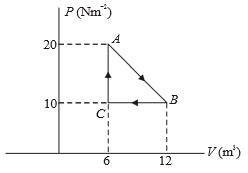1.  Zero

2.  90 J

3.  30 J

4.  60 J

Concept Questions :-

Work done by gas
High Yielding Test Series + Question Bank - NEET 2020

Difficulty Level:

If 32 gm of ${O}_{2}$ at $27°\mathrm{C}$ is mixed with 64 gm of ${\mathrm{O}}_{2}$ at $327°\mathrm{C}$ in an adiabatic vessel, then the final temperature of the mixture will be :

1.  $200°\mathrm{C}$

2.  $227°\mathrm{C}$

3.  $314.5°\mathrm{C}$

4.  $235.5°\mathrm{C}$

Concept Questions :-

Molar Specific heat
High Yielding Test Series + Question Bank - NEET 2020

Difficulty Level:

If ${W}_{1}$ is the work done in compressing an ideal gas from a given initial state through a certain volume isothermally and ${W}_{2}$ is the work done in compressing the same gas from the same initial state through the same volume adiabatically, then:

(1) ${W}_{1}={W}_{2}$

(2) ${W}_{1}<{W}_{2}$

(3) ${W}_{1}>{W}_{2}$

(4) ${W}_{1}=2{W}_{2}$

Concept Questions :-

Work done by gas
High Yielding Test Series + Question Bank - NEET 2020

Difficulty Level:

An ideal gas changes from state 'a' to state 'b' as shown in figure. What is the work done by the gas in the process?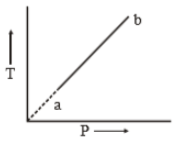1. zero

2. positive

3. negative

4. infinite

Concept Questions :-

Work done by gas
High Yielding Test Series + Question Bank - NEET 2020

Difficulty Level: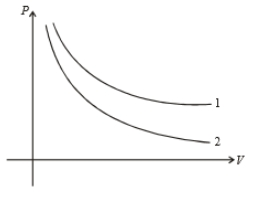P-V graph of two gases during adiabatic processes are shown in figure. Plots 1 and 2 should correspond, respectively to :

1. He, ${\mathrm{O}}_{2}$

2. ${\mathrm{O}}_{2}$ and He

3. He and Ar

4. ${\mathrm{O}}_{2}$ and ${\mathrm{N}}_{2}$

Concept Questions :-

Molar Specific heat
High Yielding Test Series + Question Bank - NEET 2020

Difficulty Level:

For an ideal gas V-T curves at constant pressure ${\mathrm{P}}_{1}$ and ${\mathrm{P}}_{2}$ are shown in figure. From the figure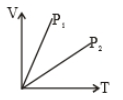1. ${\mathrm{P}}_{1}>{\mathrm{P}}_{2}$

2. ${\mathrm{P}}_{1}<{\mathrm{P}}_{2}$

3. ${\mathrm{P}}_{1}={\mathrm{P}}_{2}$

4. ${\mathrm{P}}_{1}\le {\mathrm{P}}_{2}$

Concept Questions :-

Types of processes
High Yielding Test Series + Question Bank - NEET 2020

Difficulty Level:

During an experiment and ideal gas is found to obey an additional law $V{P}^{2}$=constant. The gas is initially at a temperature T and volume V. When it expand to a volume 2V, the temperature becomes.

(1) T

(2) 2T

(3) T$\sqrt{2}$

(4) T/2

Concept Questions :-

Types of processes
High Yielding Test Series + Question Bank - NEET 2020

Difficulty Level:

The P-V diagram of a certain process (Carnot cycle) is as shown in the figre. The process is represented as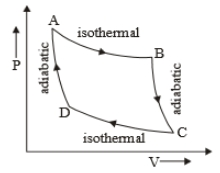1.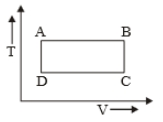2.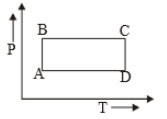3.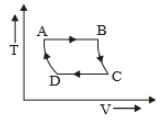4.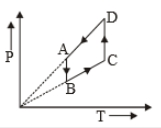Concept Questions :-

Types of processes